# #冲刺创作新星#图像金字塔原理与轮廓识别检测 原创

0收藏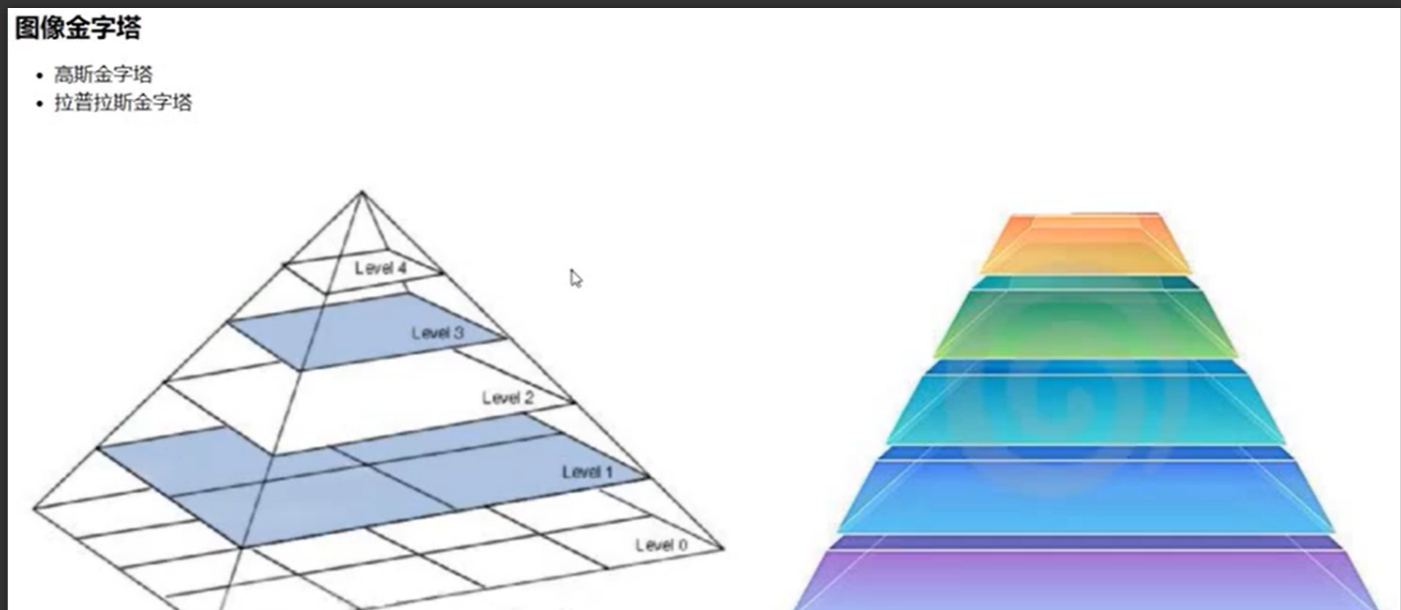### 高斯金字塔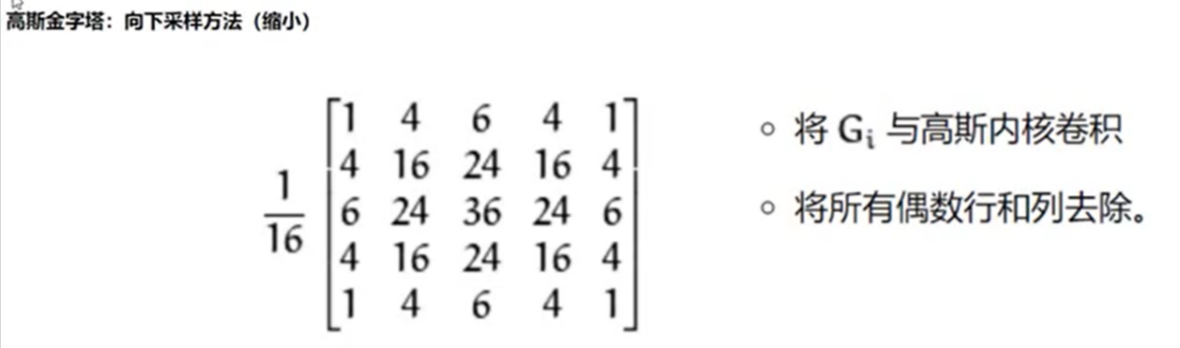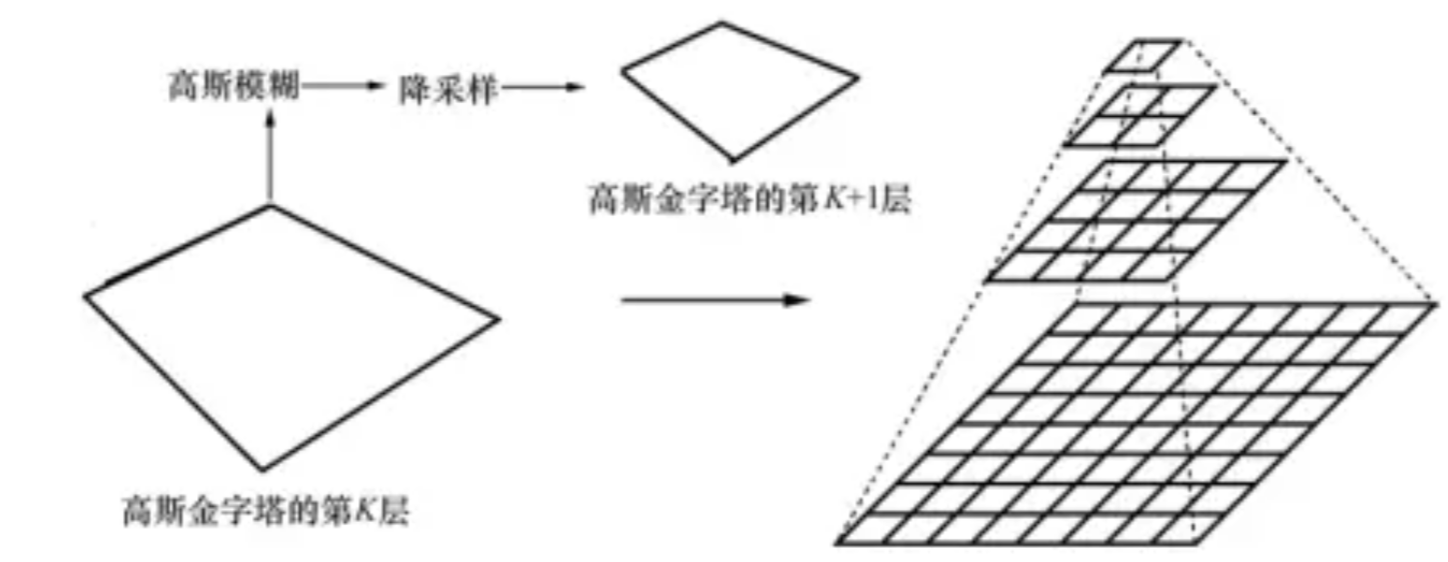### 函数介绍

up = cv2.pyrUp(img)：高斯放大函数，传入需要放大的图片即可。

down = cv2.pyrDown(up)：高斯缩小函数，传入需要放大的图片即可。

### 代码实例

``````import cv2
import numpy as np
import matplotlib.pyplot as plt
def cv2_imshow(name,img):#构造展示图像的函数
cv2.imshow(name,img)
cv2.waitKey(0)
cv2.destroyAllWindows()
cv2_imshow("XY",img)#展示原图像
up = cv2.pyrUp(img)#进行高斯放大
cv2_imshow("up",up)#展示图像
down = cv2.pyrDown(up)#进行高斯缩小
cv2_imshow("down",down)#展示图像``````

### 运行结果### 拉普拉斯金字塔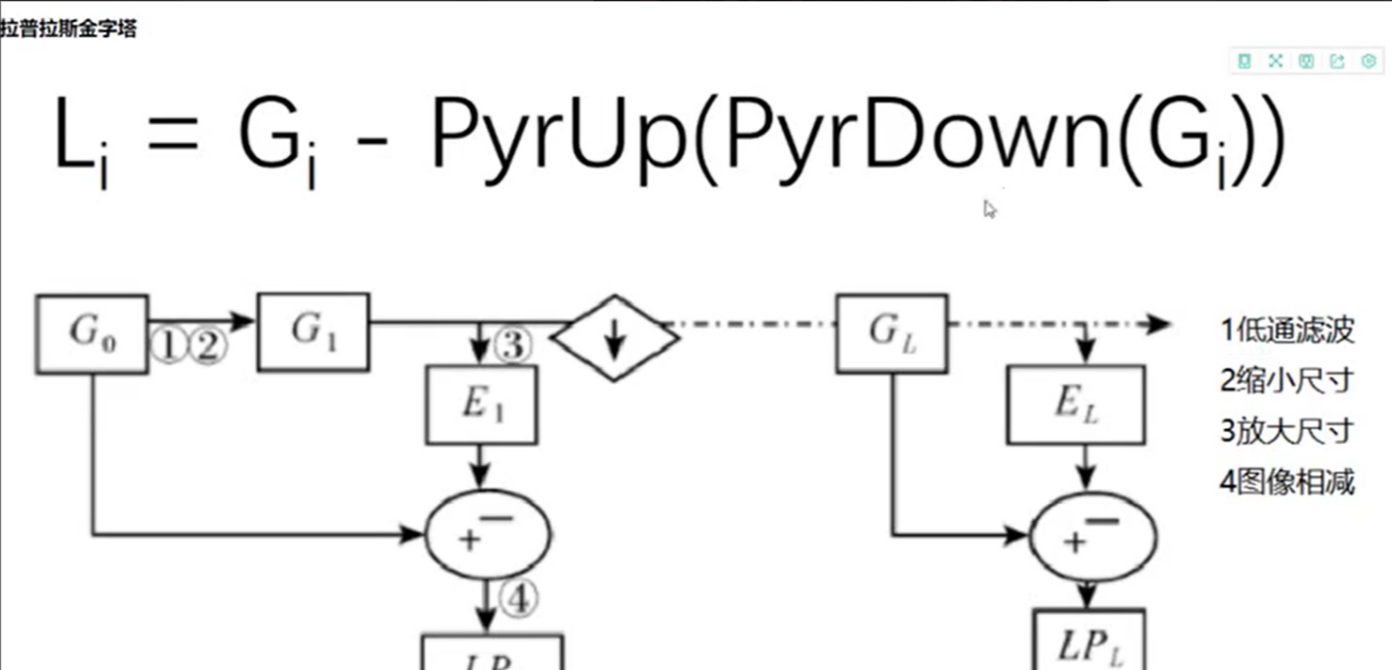### 代码实例

``````import cv2
import numpy as np
import matplotlib.pyplot as plt
def cv2_imshow(name,img):#构造展示图像的函数
cv2.imshow(name,img)
cv2.waitKey(0)
cv2.destroyAllWindows()
cv2_imshow("XY",img)#展示原图像
down = cv2.pyrDown(img)#高斯缩小
down_up = cv2.pyrUp(down)#高斯放大
down_up = cv2.resize(down_up,(220,165))#调整图像大小
laplacian = img - down_up#拉普拉斯金字塔，相当于在求图像残差
cv2_imshow("laplacian",laplacian)#展示图像``````### 图像轮廓检测

contours,hierarchy = cv2.findContours(thresh,mode,method):这里的thresh是进行过二值化处理后需要进一步检测轮廓的图像，mode参数是检索模式，method则是轮廓逼近方法的选择，一般我们选择第一个，第二个是当处理时间过长时采用的，一般我们处理的图像图像都比较简单。

contours：是带有轮廓信息的列表（可以根据层级取出不同的轮廓信息）。

hierarchy：如果输入选择cv2.RETR_TREE，则以树形结构组织输出，hierarchy的四列分别对应下一个轮廓编号、上一个轮廓编号、父轮廓编号、子轮廓编号，该值为负数表示没有对应项。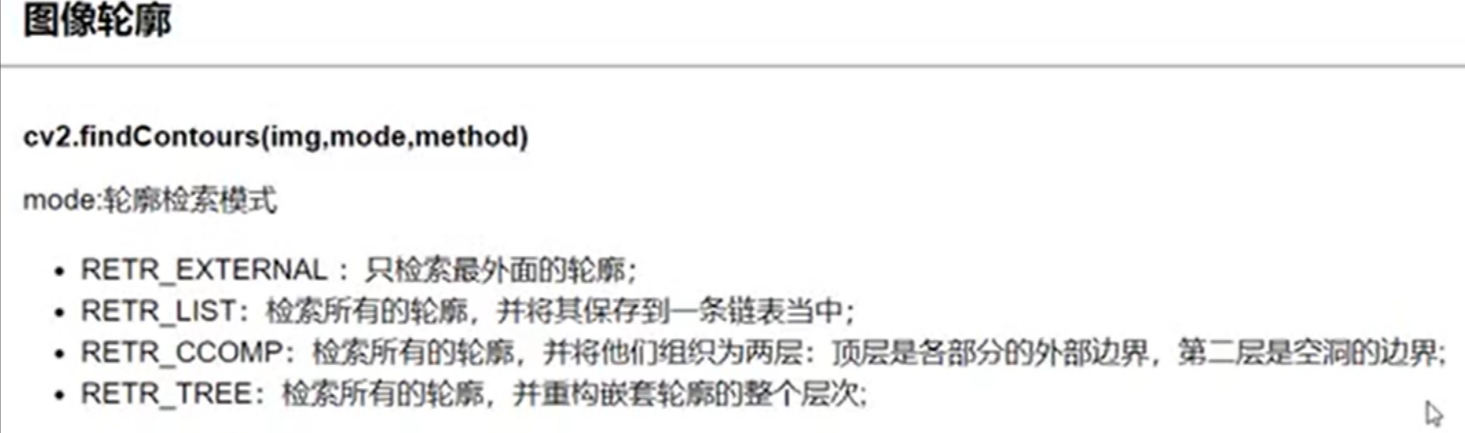res = cv2.drawContours(draw_img,contours,-1,(0,0,255)，1)：画出图像轮廓，draw_img参数是导入要画轮廓信息的图像（可以是彩色图，但是要注意，图像画上轮廓之后就会被保存，无法恢复，因此我们传入的图像应该先备份），contours是该图像的轮廓信息，-1表示画出所有轮廓，0则表示画出第0个轮廓（以此类推），(0,0,255)表示要画的轮廓颜色，1是表示要画的轮廓粗细。

cnt = contours：对处理图像的轮廓进行选定，以进行更有针对性的操作。

x,y,w,h = cv2.boundingRect(cnt)：计算出该轮廓所在的矩形，返回值x,y,w,h分别表示矩形左上角的横纵坐标以及图像的宽度和高度。

cv2.rectangle(img,(x,y),(x+w,y+h),(0,255,0),2)：根据上一个函数得到的返回值画出矩形，img是要画矩形的图像，(x,y)为矩形左上角坐标，（x+w,y+h)为右下角坐标，(0,255,0)是矩形的颜色，2则是矩形线条的宽度

area = cv2.contourArea(cnt)：能够计算指定轮廓的面积。

extent = float(area)/rect_area：计算指定轮廓与矩形的面积比。

cv2.arcLength(cnt,True)：计算周长，True表示该轮廓曲线闭合，False则表示轮廓曲线断开。

``````import cv2
import numpy as np
import matplotlib.pyplot as plt
def cv2_imshow(name,img):#构造展示图像的函数
cv2.imshow(name,img)
cv2.waitKey(0)
cv2.destroyAllWindows()
cv2_imshow("img",img)#展示原图
img_gray = cv2.cvtColor(img,cv2.COLOR_BGR2GRAY)#转化为灰度图
ret,thresh = cv2.threshold(img_gray,120,255,cv2.THRESH_BINARY)#进行二值化处理
cv2_imshow("thresh",thresh)#展示二值化处理后的图像
contours,hierarchy = cv2.findContours(thresh,cv2.RETR_TREE,cv2.CHAIN_APPROX_NONE)#这个版本只返回两个值,轮廓以及轮廓的层级
draw_img = img.copy()#复制一下原图
res = cv2.drawContours(draw_img,contours,-1,(0,0,255))#-1表示画出全部轮廓，可以单独画出某个轮廓
cv2_imshow("res",res)#展示图像
#轮廓特征（面积与周长）
cnt = contours#指定轮廓
area = cv2.contourArea(cnt)#计算面积
perimeter = cv2.arcLength(cnt,True)#计算周长，True表示该图像曲线闭合``````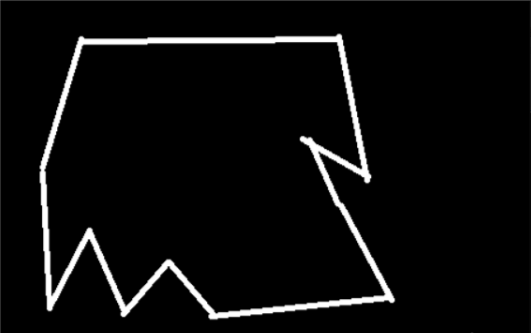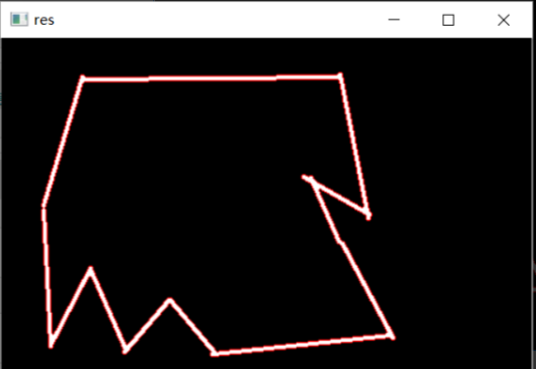``````import cv2
import numpy as np
import matplotlib.pyplot as plt
def cv2_imshow(name,img):
cv2.imshow(name,img)
cv2.waitKey(0)
cv2.destroyAllWindows()
cv2_imshow("img",img)#展示原图像
img_gray = cv2.cvtColor(img,cv2.COLOR_BGR2GRAY)#转化为灰度图
ret,thresh = cv2.threshold(img_gray,127,255,cv2.THRESH_BINARY)#转化为二值图像
contours,hierarchy = cv2.findContours(thresh,cv2.RETR_TREE,cv2.CHAIN_APPROX_NONE)#计算轮廓
cnt = contours#选定轮廓，可以自由选择，改为1，2，3等等都可以
x,y,w,h = cv2.boundingRect(cnt)#计算矩形的左上点坐标，图片的高和宽（不一定是正数）
cv2.rectangle(img,(x,y),(x+w,y+h),(0,255,0),2)#给出左上点的和右下点矩形坐标计算出矩形的大小和位置
cv2_imshow("img",img)#展示画好矩形的图像
area = cv2.contourArea(cnt)#计算轮廓面积
rect_area = w*h
extent = float(area)/rect_area#计算轮廓与矩形的面积比
print("轮廓与矩形的面积比为：",extent)``````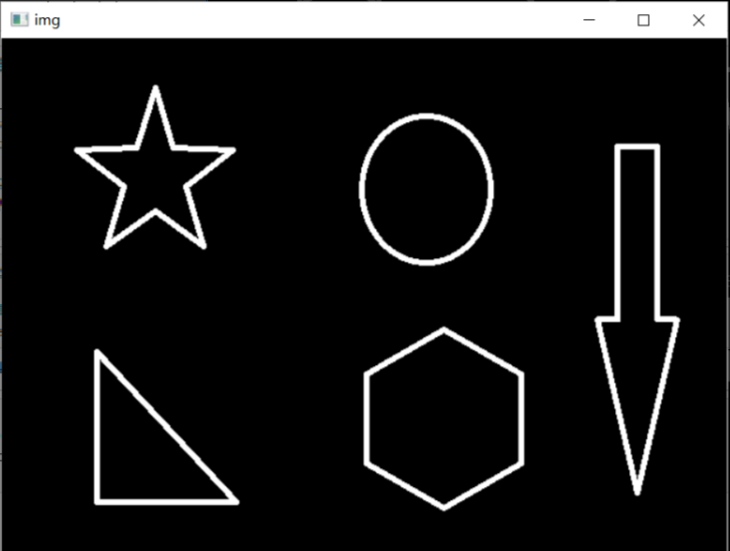画好矩形后的图像（可以自由选择轮廓，这里选的是0）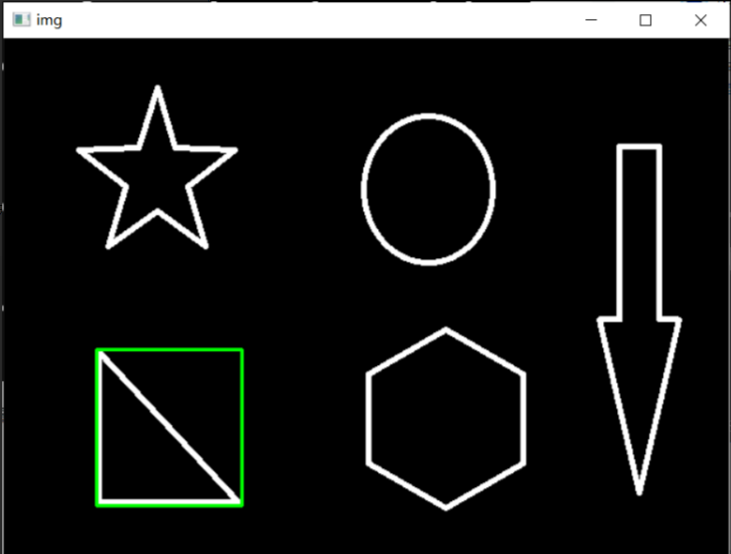图像模板检测匹配

Opencv提供了cv2.matchTemplate()函数进行简单的图像检测识别，它的原理是读入一张要进行匹配的单通道图像，再读入一张从模板上截下来的单通道图片模板进行匹配，通过将模板在图像上一格一格的进行移动，最后比对出像素最接近的模板区域。该函数使用十分便捷，但通常需要结合其他识别方式使用。

res = cv2.matchTemplate(img,template,1)：img是要进行匹配的图像，template是模板，1表示识别方式，当然也可以写出具体的参数名，识别方式种类如下。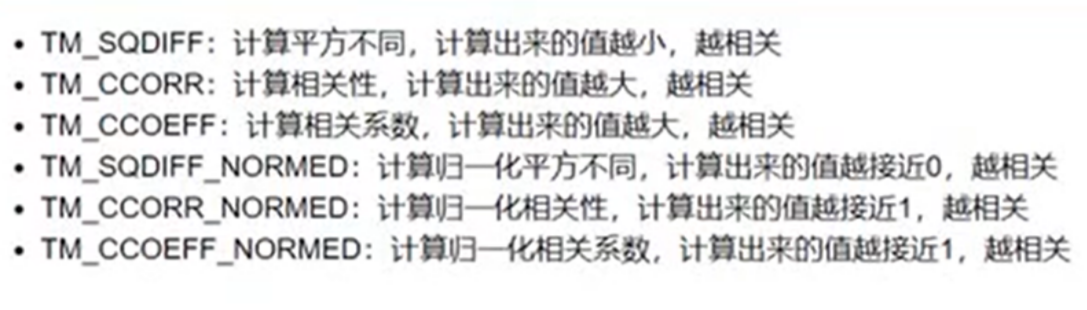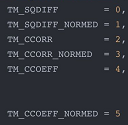res是一个矩阵，它记录了模板在图像上每移动一次像素点所计算得到的平方差或者相关系数，我们可以通过min_val,max_val,min_loc,max_loc = cv2.minMaxLoc(res)函数来得到它的最大值和最小值以及相对应的坐标，然后在那个位置画出矩形，我们就成功的把检测结果画在了图像上。

``````import cv2
import numpy as np
import matplotlib.pyplot as plt
def cv2_imshow(name,img):
cv2.imshow(name,img)
cv2.waitKey(0)
cv2.destroyAllWindows()
res = cv2.matchTemplate(img,template,1)#img为要检测的图片，template为模板，1表示检测方式
min_val,max_val,min_loc,max_loc = cv2.minMaxLoc(res)#得到最大最小值及其坐标
h,w = template.shape[:2]#得到模板的高和宽用以确定矩形大小
methods = ['cv2.TM_CCOEFF',"cv2.TM_CCOEFF_NORMED","cv2.TM_CCORR","cv2.TM_CCORR_NORMED","cv2.TM_SQDIFF","cv2.TM_SQDIFF_NORMED"]
for i in methods:
draw_img = img.copy()#复制图像
method = eval(i)
res = cv2.matchTemplate(img,template,method)#模板匹配操作
min_val,max_val,min_loc,max_loc = cv2.minMaxLoc(res)#计算出最大值坐标和最小值坐标
if i in ["cv2.TM_SQDIFF","cv2.TM_SQDIFF_NORMED"]:#判断是否进行归一化操作，寻找匹配的坐标
top_left = min_loc#如果进行了归一化操作，则应选择最小值
else:
top_left = max_loc#如果未进行归一化操作，则选择最大值
bottom_right = (top_left+w,top_left+h)#求出右下角矩形坐标
cv2.rectangle(draw_img,top_left,bottom_right,255,2)#计算矩形的左上点坐标，图片的高和宽（不一定是正数）
plt.subplot(121),plt.imshow(res,cmap = 'gray')
plt.xticks([]),plt.yticks([])#隐藏坐标轴
plt.subplot(122),plt.imshow(draw_img,cmap = 'gray')
plt.xticks([]),plt.yticks([])#隐藏坐标轴
plt.suptitle(i)#显示标题``````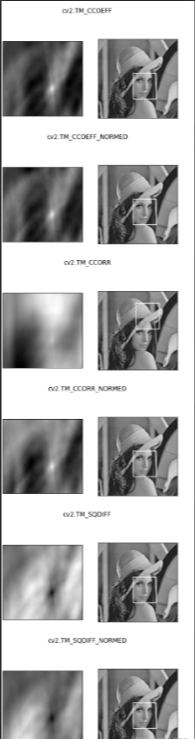### 天天酷跑中的金币识别

``````import cv2
import numpy as np
import matplotlib.pyplot as plt
def cv2_imshow(name,img):
cv2.imshow(name,img)
cv2.waitKey(0)
cv2.destroyAllWindows()
cv2_imshow("gray",img_gray)
res = cv2.matchTemplate(img_gray,template,cv2.TM_CCOEFF_NORMED)
h,w = template.shape[:2]
threshold = 0.5
#取匹配程度大于50%的坐标
loc = np.where(res>threshold)
for pt in zip(*loc[::-1]):
bottom_right = (pt+w,pt+h)
cv2.rectangle(img_rgb,pt,bottom_right,(0,225,0),1)
cv2_imshow("img_rgb",img_rgb)``````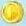1帖子
视频
声望
粉丝
相关问题
社区精华内容